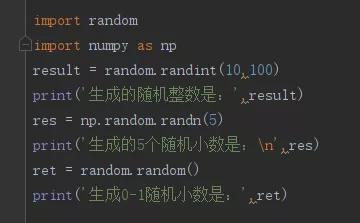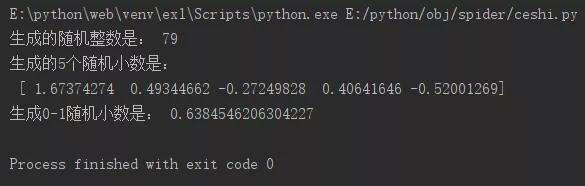• 生成随机数 使用 random 模块 random.random() 用于随机生成一个0到1的浮点数 random.randint(start,stop) 随机生成[start,stop]区间内的整数 代码示例： import random print (random.random()) print(random....
生成随机数
使用 random 模块
random.random() 用于随机生成一个0到1的浮点数
random.randint(start,stop) 随机生成[start,stop]区间内的整数
代码示例：
import random
print (random.random())
print(random.randint(2,5))

输出结果：
0.28113894170242715
2
生成随机矩阵
import numpy as np

print(np.random.rand(4,5))
print(np.random.randint(2,4,(3,3)))#(3,3)表示矩阵大小

[[0.9301374  0.3101388  0.87523224 0.72690872 0.29985264]
[0.83968348 0.62680457 0.80127134 0.22433064 0.01149985]
[0.2193549  0.25525533 0.96211193 0.33978737 0.06699431]
[0.05281168 0.07236824 0.0226833  0.99250086 0.72282644]]
[[3 2 3]
[2 3 3]
[3 2 3]]


展开全文• 原标题：「每日一练」巧用python生成随机数随机数在我们的生产和生活中有很多的应用场景，比如说登录验证的随机数字等等，那么你知道在Python中怎么生成随机数吗？往下看，就是这么简单！题目python中生成随机整数、...
原标题：「每日一练」巧用python生成随机数随机数在我们的生产和生活中有很多的应用场景，比如说登录验证的随机数字等等，那么你知道在Python中怎么生成随机数吗？往下看，就是这么简单！题目python中生成随机整数、随机小数、0--1之间小数方法代码先上代码~运行效果编辑题目详述程序分析：随机整数：random.randint(a,b),生成区间内的整数随机小数：习惯用numpy库，利用np.random.randn(5)生成5个随机小数0-1随机小数：random.random(),括号中不传参第一行：import randomimport numpy as np分别引用random和numpy库进行调用第二行：result = random.randint(10,100)print('生成的随机整数是：',result)使用random的randint方法，注意里边需要传递两个参数，用来表示生成在哪两个整数之间的随机数第三行：res = np.random.randn(5)print('生成的5个随机小数是：\n',res)利用np.random.randn(5)生成5个随机小数，并且将它打印出来，其中\n是Python里边的换行符第四行：ret = random.random()print('生成0-1随机小数是：',ret)random.random()生成0-1之间的随机小数，括号中不传参，生成的随机数再乘以一个值，就得到了一个扩大的范围，这个经常会用到进阶:轻松的就实现想要的目的是不是很便捷呢结束语总体来说思路只要有了，以后遇到类似的问题就可以解决了，是不是发现原来Python可以这么神奇！———— e n d ————觉得文章不错的，欢迎点在看和转发返回搜狐，查看更多责任编辑：
展开全文• 1. 使用函数 np.random.random由于 np.random.random() 默认生成 0~1 之间的小数，因此需要转换一下如生成 3*3 的 -1~1 之间的随机数矩阵-1 + 2*np.random.random((3,3))# -*- coding:utf-8 -*-import matplotlib....
1. 使用函数 np.random.random由于 np.random.random() 默认生成 0~1 之间的小数，因此需要转换一下如生成 3*3 的 -1~1 之间的随机数矩阵-1 + 2*np.random.random((3,3))# -*- coding:utf-8 -*-import matplotlib.pyplot as pltimport pylabimport cv2import numpy as npimg = plt.imread("1.png")      #在这里读取图片#plt.imshow(img)          #显示读取的图片#pylab.show()print "start processing..."for i in range(1,200):# fil = np.random.randint(0, 10, size=[3, 3])fil = -1 + 2*np.random.random((3,3)) res = cv2.filter2D(img,-1,fil)      #使用opencv的卷积函数# plt.imshow(res)          #显示卷积后的图片pic_name = str(i) + ".png"# plt.imsave(pic_name, res)# plt.imsave("res.jpg",res)# pylab.show()print "complete!"以上这篇Python 生成 -1~1 之间的随机数矩阵方法就是小编分享给大家的全部内容了，希望能给大家一个参考，也希望大家多多支持我们。时间： 2018-08-02
展开全文• ## 生成3行4列整数矩阵 rand_data = np.random.randint(1, 10, (3, 4)) rand_data ## Out:array([[5, 5, 3, 6], ## [4, 7, 3, 7], ## [5, 2, 1, 6]]) ## shuffle(x) np.random.shuffle(rand_data) rand_data #...python
• import random mu=np.array([[0, 100],[-100, 900]]) sigma=np.array([[1, 1],[1, 1]]) number=random.normalvariate(mu, s) mu array([[ 0, 100], [-100, 900]]) sigma array([[1, 1], [1, 1]]) ...
import random
mu=np.array([[0, 100],[-100, 900]])
sigma=np.array([[1, 1],[1, 1]])
number=random.normalvariate(mu, s)
mu
array([[   0,  100],
[-100,  900]])
sigma
array([[1, 1],
[1, 1]])
number
array([[-5.89493150e-01,  9.94105068e+01],
[-1.00589493e+02,  8.99410507e+02]])

对应位置组队


展开全文• 编写一个Python函数，生成一个m行n列的元素值不大于20的随机数矩阵（二维列表）。 提示：使用random.randint()函数来生成随机数。 函数接口定义： def generateMatrix(m,n) m: 行数，int, 1 < m < 100; n: ...列表 算法
• 为了理解机器学习中的统计方法，你必须了解机器学习中随机性的来源，即一种叫做伪随机数生成器的数学工具。在本教程中，你将了解伪随机数生成器，以及何时在机器学习中控制随机性，或用随机性来进行控制。学完这篇...
• 最近在听北邮陈光老师在B站的《python编程与实践》《Python编程与实践》第二课 by 北邮陈光课程是面向初学者的，不过我很喜欢陈老师在开课之前的一些方法论。 以下皆是陈老师课程中给出学习语言的建议： 多思考【多...numpy
• ### random.seed(1) 设置种子seed,可以每次生成相同的随机整数 ### random.randint(a,b) 生成1个在a至b区间的随机整数 print(random.randint(0,10)) print('.........\n') ### random.randint(a,b,size=n) 生成n个在...
• 今天小编就为大家分享一篇Python 生成 -1~1 之间的随机数矩阵方法，具有很好的参考价值，希望对大家有所帮助。一起跟随小编过来看看吧
• 生成随机矩阵 用numpy包实现 生成随机整数矩阵 numpy.random.randint(low[,high,size]) 值范围位于半区间[low,high)中 其他函数 np.random.rand(size) 返回[0, 1)之间的随机浮点数矩阵。size为int型。 np.random....
• 本系类笔记采用的是Python3.5X版本，编程环境为Windows64位下的Anaconda所有代码部分均为连续的，“结果”为在jupyter分步运行结果代码部分：import numpy as npsample1 = np.random.random((3,2))#生成3行2列从0到1...
• ## Python中随机数的生成

万次阅读 多人点赞 2018-06-05 16:50:42
Python中可以用于随机数生成的有两种主要途径，一是random模块，另一个...random模块中将近有7个函数都是可以用来生成随机数的： ① random.random() 功能：随机生成一个 [0,1) 的浮点数 用法： import rando......

# python生成随机数矩阵python 订阅